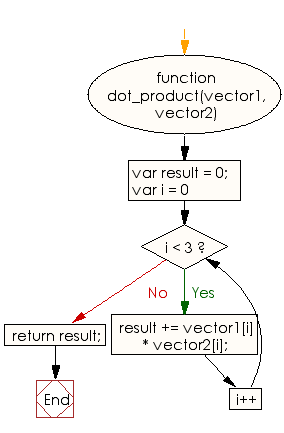# JavaScript: Create the dot products of two given 3D vectors

## JavaScript Basic: Exercise-108 with Solution

Write a JavaScript program to create the dot products of two given 3D vectors.

Note: The dot product is the sum of the products of the corresponding entries of the two sequences of numbers.

Sample Solution:

HTML Code:

``````<!DOCTYPE html>
<html>
<meta charset="utf-8">
<meta name="viewport" content="width=device-width">
<title> Create the dot products of two given 3D vectors</title>
<body>

</body>
</html>
```
```

JavaScript Code:

``````function dot_product(vector1, vector2) {
var result = 0;
for (var i = 0; i < 3; i++) {
result += vector1[i] * vector2[i];
}
return result;
}
console.log(dot_product([1,2,3], [1,2,3]))
console.log(dot_product([2,4,6], [2,4,6]))
console.log(dot_product([1,1,1], [0,1,-1]))
``````

Sample Output:

```14
56
0
```

Flowchart:ES6 Version:

``````function dot_product(vector1, vector2) {
let result = 0;
for (let i = 0; i < 3; i++) {
result += vector1[i] * vector2[i];
}
return result;
}
console.log(dot_product([1,2,3], [1,2,3]))
console.log(dot_product([2,4,6], [2,4,6]))
console.log(dot_product([1,1,1], [0,1,-1]))
``````

Live Demo:

See the Pen javascript-basic-exercise-109 by w3resource (@w3resource) on CodePen.

What is the difficulty level of this exercise?

Test your Programming skills with w3resource's quiz.

﻿

## JavaScript: Tips of the Day

Checks if a string is an anagram of another string (case-insensitive, ignores spaces, punctuation and special characters)

Example:

```const isAnagram = (str1, str2) => {
const normalize = str =>
str
.toLowerCase()
.replace(/[^a-z0-9]/gi, '')
.split('')
.sort()
.join('');
return normalize(str1) === normalize(str2);
};
console.log(isAnagram('iceman', 'cinema')); // true
```

Output:

```true
```